# Geometry Mathematics 2 Set A 2015-2016 SSC (English Medium) 10th Standard Board Exam Question Paper Solution

Geometry Mathematics 2 [Set A]
Date & Time: 10th March 2016, 11:00 am
Duration: 2h

 1 | Solve any five sub-questions
 1.1

ΔDEF ~ ΔMNK. If DE = 5, MN = 6, then find the value of "A(ΔDEF)"/"A(ΔMNK)"

Concept: Similar Triangles
Chapter: [0.01] Similarity
 1.2

In the following figure, in ΔABC, ∠B = 90°, ∠C = 60°, ∠A = 30°, AC = 18 cm. Find BC.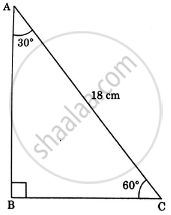Concept: Property of 30°- 60°- 90° Triangle Theorem
Chapter: [0.02] Pythagoras Theorem
 1.3

In the following figure, m(arc PMQ) = 130o, find ∠PQS.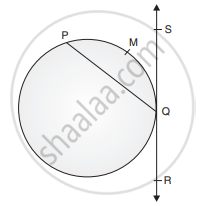Concept: Angle Subtended by the Arc to the Point on the Circle
Chapter: [0.03] Circle
 1.4

If the angle θ= –60º, find the value of cosθ.

Concept: Trigonometric Ratios of Complementary Angles
Chapter: [0.06] Trigonometry
 1.5

Find the slope of the line with inclination 30° .

Concept: Slope of a Line
Chapter: [0.05] Co-ordinate Geometry
 1.6

Using Euler’s formula, find V if E = 30, F = 12.

Concept: Euler's Formula
Chapter: [0.07] Mensuration
 2 | Solve any four sub-questions:
 2.1

In the following figure, in ΔPQR, seg RS is the bisector of ∠PRQ. If PS = 6, SQ = 8, PR = 15, find QR.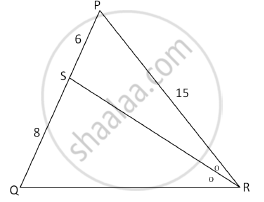Concept: Similarity of Triangles
Chapter: [0.01] Similarity
 2.2

In the following figure, a tangent segment PA touching a circle in A and a secant PBC is shown. If AP = 15, BP = 10, find BC.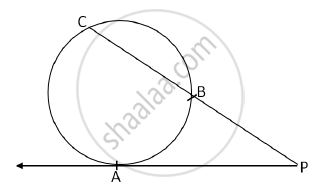Concept: Tangent - Secant Theorem
Chapter: [0.03] Circle
 2.3

Draw an equilateral ΔABC with side 6.2 cm and construct its circumcircle

Concept: Construction of Similar Triangle
Chapter: [0.04] Geometric Constructions
 2.4

For the angle in standard position if the initial arm rotates 25° in anticlockwise direction, then state the quadrant in which terminal arm lies (Draw the figure and write the answer).

Concept: Angles in Standard Position
Chapter: [0.06] Trigonometry
 2.5

Find the area of sector whose arc length and radius are 10 cm and 5 cm respectively

Concept: Areas of Sector and Segment of a Circle
Chapter: [0.07] Mensuration
 2.6

Find the surface area of a sphere of radius 4.2 cm. (π = 22/7)

Concept: Surface Area and Volume of Three Dimensional Figures
Chapter: [0.07] Mensuration
 3 | Solve any three sub-questions:
 3.1

Adjacent sides of a parallelogram are 11 cm and 17 cm. If the length of one of its diagonal is 26 cm, find the length of the other.

Concept: Apollonius Theorem
Chapter: [0.02] Pythagoras Theorem
 3.2

In the following figure, secants containing chords RS and PQ of a circle intersects each other in point A in the exterior of a circle if m(arc PCR) = 26°, m(arc QDS) = 48°, then find:
(i) m∠PQR
(ii) m∠SPQ
(iii) m∠RAQ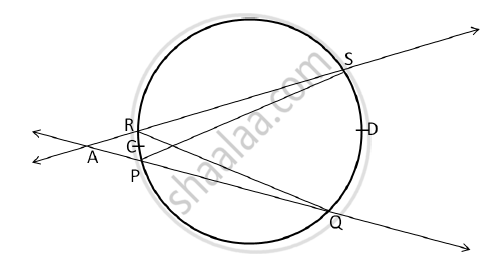Concept: Angle Subtended by the Arc to the Point on the Circle
Chapter: [0.03] Circle
 3.3

Draw a circle of radius 3.5 cm. Take any point K on it. Draw a tangent to the circle at K without using centre of the circle.

Concept: Construction of a Tangent to the Circle at a Point on the Circle
Chapter: [0.04] Geometric Constructions
 3.4

If sec alpha=2/sqrt3  , then find the value of (1-cosecalpha)/(1+cosecalpha) where α is in IV quadrant.

Concept: Trigonometric Identities
Chapter: [0.06] Trigonometry
 3.5

Write the equation of the line passing through the pair of points (2, 3) and (4, 7) in the form of y = mx + c.

Concept: General Equation of a Line
Chapter: [0.05] Co-ordinate Geometry
 4 | Solve any two sub-questions:
 4.1

Prove that “The lengths of the two tangent segments to a circle drawn from an external point are equal.”

Concept: Number of Tangents from a Point on a Circle
Chapter: [0.03] Circle
 4.2

A person standing on the bank of river observes that the angle of elevation of the top of a tree standing on the opposite bank is 60°. When he moves 40 m away from the bank, he finds the angle of elevation to be 30°. Find the height of the tree and width of the river. (sqrt 3=1.73)

Concept: Heights and Distances
Chapter: [0.06] Trigonometry
 4.3

A (5, 4), B (-3, -2) and C (1, -8) are the vertices of a triangle ABC. Find the equations of the median AD and line parallel to AC passing through the point B.

Concept: General Equation of a Line
Chapter: [0.05] Co-ordinate Geometry
 5 | Solve any two sub-questions:
 5.1

In the following figure, AE = EF = AF = BE = CF = a, AT ⊥ BC. Show that AB = AC =  sqrt3xxa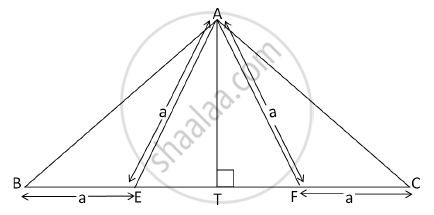Concept: Similarity in Right Angled Triangles
Chapter: [0.02] Pythagoras Theorem
 5.2

ΔSHR ~ ΔSVU. In ΔSHR, SH = 4.5 cm, HR = 5.2 cm, SR = 5.8 cm and "SH"/("SV")=3/5. Construct ΔSVU.

Concept: Basic Geometric Constructions
Chapter: [0.04] Geometric Constructions
 5.3

Water flows at the rate of 15 m per minute through a cylindrical pipe, having the diameter 20 mm. How much time will it take to fill a conical vessel of base diameter 40 cm and depth 45 cm?

Concept: Surface Area and Volume of Different Combination of Solid Figures
Chapter: [0.07] Mensuration

#### Request Question Paper

If you dont find a question paper, kindly write to us

View All Requests

#### Submit Question Paper

Help us maintain new question papers on Shaalaa.com, so we can continue to help students

only jpg, png and pdf files

## Maharashtra State Board previous year question papers 10th Standard Board Exam Geometry Mathematics 2 with solutions 2015 - 2016

Maharashtra State Board 10th Standard Board Exam Geometry Maths 2 question paper solution is key to score more marks in final exams. Students who have used our past year paper solution have significantly improved in speed and boosted their confidence to solve any question in the examination. Our Maharashtra State Board 10th Standard Board Exam Geometry Maths 2 question paper 2016 serve as a catalyst to prepare for your Geometry Mathematics 2 board examination.
Previous year Question paper for Maharashtra State Board 10th Standard Board Exam Geometry Maths 2-2016 is solved by experts. Solved question papers gives you the chance to check yourself after your mock test.
By referring the question paper Solutions for Geometry Mathematics 2, you can scale your preparation level and work on your weak areas. It will also help the candidates in developing the time-management skills. Practice makes perfect, and there is no better way to practice than to attempt previous year question paper solutions of Maharashtra State Board 10th Standard Board Exam.

How Maharashtra State Board 10th Standard Board Exam Question Paper solutions Help Students ?
• Question paper solutions for Geometry Mathematics 2 will helps students to prepare for exam.
• Question paper with answer will boost students confidence in exam time and also give you an idea About the important questions and topics to be prepared for the board exam.
• For finding solution of question papers no need to refer so multiple sources like textbook or guides.